# SAT Math Multiple Choice Question 991: Answer and Explanation

### Test Information

Question: 991

11. If the longer leg of a right triangle has length 32 centimeters, and the measure ofthe angle that is adjacent to that leg is 30°, which of the following represents thelength, in centimeters, of the hypotenuse of the triangle?

• A.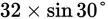• B.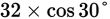• C.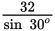• D.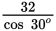Explanation:

D

Difficulty: Medium

Category: Additional Topics in Math / Trigonometry

Strategic Advice: Take a peek at the answer choices-they involve trig functions, so set up a proportion using SOH CAH TOA.

Getting to the Answer: Draw a quick sketch of a right triangle. Label the longer leg 32 and the angle adjacent to it 30°: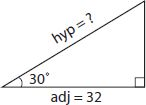Notice that you have the adjacent side (32) and you're looking for the hypotenuse, so use cosine: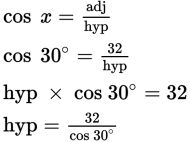This matches (D).